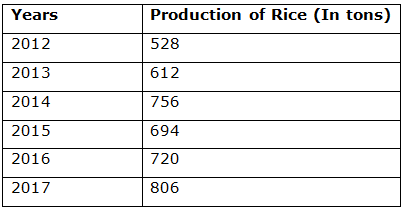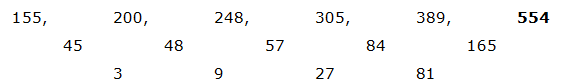# CWC/FCI Prelims 2019 – Quantitative Aptitude Questions (Day-26)

Dear Readers, Exam Race for the Year 2019 has already started, To enrich your preparation here we are providing new series of Practice Questions on Quantitative Aptitude – Section for CWC/FCI Exam. Aspirants, practice these questions on a regular basis to improve your score in aptitude section. Start your effective preparation from the right beginning to get success in upcoming CWC/FCI Exam.

[WpProQuiz 5703]

Directions (Q. 1 – 5): Find the Missing term in the following number series?

1) 208, 104, 156, 390, ? , 6142.5

a) 1450

b) 2175

c) 1365

d) 2890

e) 3345

2) 155, 200, 248, 305, 389, ?

a) 554

b) 478

c) 512

d) 596

e) 620

3) 45, 48, 102, 315, 1272, ?

a) 5125

b) 5530

c) 6145

d) 6375

e) 4560

4) 42, 22, 30, 87, ? , 3421

a) 536

b) 412

c) 624

d) 748

e) 856

5) 7020, 3510, 1170, 292.5, ? , 9.75

a) 106.25

b) 76.5

c) 58.5

d) 62.5

e) 95.25

Directions (Q. 6 – 10): Study the following information carefully and answer the given questions:

The following table shows the total production of rice (In tons) in 5 different years in a certain state.6) Find the difference between the total production of rice in the year 2012 and 2014 together to that of total production of rice in the year 2015 and 2017 together?

a) 216

b) 278

c) 324

d) 192

e) None of these

7) There are 3 categories (A1, A2 and A3) of rice cultivated in the state. If the ratio of production of A1, A2 and A3 category rice in the year 2013 and 2016 is 3 : 4 : 2 and 5 : 1 : 3 respectively, then find the ratio of production of A2 category rice in the year 2013 to that A3 category rice in the year 2016?

a) 25: 23

b) 37: 35

c) 12: 7

d) 17: 15

e) None of these

8) Find the average production of rice in all the given years together?

a) 715

b) 686

c) 758

d) 627

e) None of these

9) Total production of rice in the year 2014 is approximately what percentage of total production of rice in the year 2017?

a) 94 %

b) 108 %

c) 82 %

d) 75 %

e) 66 %

10) Find the difference between the average production of rice in the year 2012, 2014 and 2016 together to that of the average production of rice in the year 2013, 2015 and 2017 together?

a) 44

b) 52

c) 36

d) 68

e) None of these

Direction (1-5) :

The pattern is, *1/2, *3/2, *5/2, *7/2, *9/2,….The difference of difference is, *3

The pattern is, *1 + 3, *2 + 6, *3 + 9, *4 + 12, *5 + 15,….

The pattern is, *0.5 + 13, *1 + 23, *2 + 33, *4 + 43, *8 + 53,….

The pattern is, ÷ 2, ÷ 3, ÷ 4, ÷ 5, ÷ 6,….

Direction (6-10) :

The total production of rice in the year 2012 and 2014 together

= > 528 + 756 = 1284

The total production of rice in the year 2015 and 2017 together

= > 694 + 806 = 1500

Required difference = 1500 – 1284 = 216

Production of A2 category rice in the year 2013

= > 612*(4/9) = 272

Production of A3 category rice in the year 2016

= > 720*(3/9) = 240

Required ratio = 272: 240 = 17: 15

The average production of rice in all the given years together

= > (528 + 612 + 756 + 694 + 720 + 806)/6

= > 4116/6 = 686

Total production of rice in the year 2014 = 756

Total production of rice in the year 2017 = 806

Required % = (756/806)*100 = 94 %

The average production of rice in the year 2012, 2014 and 2016 together

= > (528 + 756 + 720)/3 = 2004/3 = 668

The average production of rice in the year 2013, 2015 and 2017 together

= > (612 + 694 + 806)/3 = 2112/3 = 704

Required difference = 704 – 668 = 36